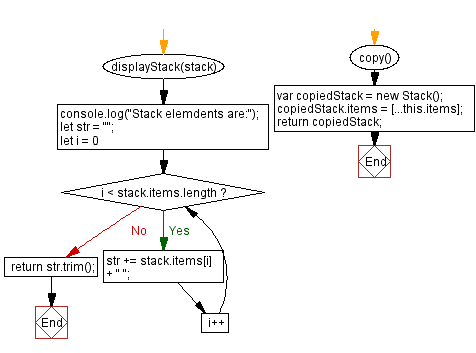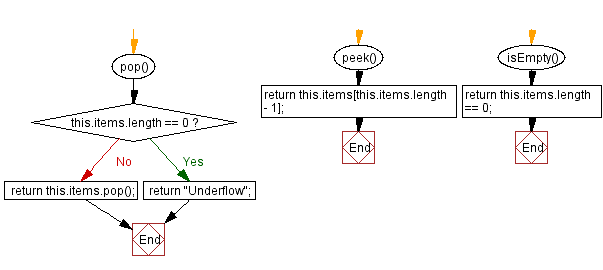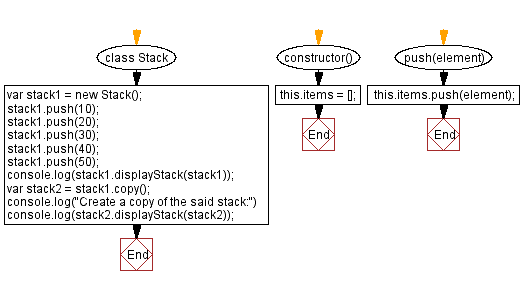# JavaScript Exercises: Create a copy of the stack

## JavaScript Stack: Exercise-25 with Solution

Write a JavaScript program to implement a stack that creates a copy of the stack.

Sample Solution:

JavaScript Code:

``````class Stack {
constructor() {
this.items = [];
}
push(element) {
this.items.push(element);
}
pop() {
if (this.items.length == 0) {
return "Underflow";
}
return this.items.pop();
}

peek() {
return this.items[this.items.length - 1];
}

isEmpty() {
return this.items.length == 0;
}
displayStack(stack) {
console.log("Stack elements are:");
let str = "";
for (let i = 0; i < stack.items.length; i++)
str += stack.items[i] + " ";
return str.trim();
}
copy() {
var copiedStack = new Stack();
copiedStack.items = [...this.items];
return copiedStack;
}
}
var stack1 = new Stack();
stack1.push(10);
stack1.push(20);
stack1.push(30);
stack1.push(40);
stack1.push(50);
console.log(stack1.displayStack(stack1));
var stack2 = stack1.copy();
console.log("Create a copy of the said stack:")
console.log(stack2.displayStack(stack2));

```
```

Sample Output:

```Stack elements are:
10 20 30 40 50
Create a copy of the said stack:
Stack elements are:
10 20 30 40 50
```

Flowchart:Live Demo:

See the Pen javascript-stack-exercise-25 by w3resource (@w3resource) on CodePen.

Improve this sample solution and post your code through Disqus

Stack Previous: Concatenates two stacks into a new stack.
Stack Exercises Next: Check if a stack is a subset of another stack.

What is the difficulty level of this exercise?

Test your Programming skills with w3resource's quiz.

﻿